# Synthesis Theory I: Final Exam

You may use any inanimate resource for this final, except a resource generated by another student during the semester such as their class notebook. Your exalted instructor is the only human you may communicate with about this exam until it is done. The exam is due by 10 a.m. sharp in room 316C next Friday. The exam may not be turned in late.

1. Soundfiles: If a soundfile is sampled at 44.1 kHz, and the soundfile is stereo and each sample is two byte (or 4 bytes for each stereo pair), then how many bytes of sound data are there in one second of sound? How many bytes are there in one minute of sound? In an hour?
 Answer: ``` 44100 samples 2 bytes ------------- * ------- * 2 channels = 176,400 bytes/sec second sample ``` ``` 176,400 bytes/sec * 60 sec/min = 10,584,000 bytes/min 10,584,000 bytes/min * 60 min/hour = 635,040,000 bytes/hour ```
2. Extra Credit: If an audio CDROM can hold 650 MB of stereo audio at a sampling rate of 44.1 kHz, and each sample is two bytes (i.e., 4 bytes for each stereo sample pair), how many seconds of audio can be stored on an audio CD? How many minutes is that?
 Answer: ```650,000,000 bytes / 4 / 44100 = 3,684.8 seconds = 61.4 minutes ``` Actually, regular CDs can hold about 74 minutes of audio. Why do CD-ROM blanks say they hold 650 MB? This is because they list the size of the CD available for computer applications which is less due to formatting, lead-in and lead-out gaps, and hopefully error-correction mechanisms. If a CD can hold 74 minutes of stereo 16-bit audio at 44.1 kHz, then this means that it can hold 783,216,000 bytes of audio data.
3. Soundfiles (2): What is this: 0xff and what does it mean?
 Answer: 0xff is the representation of a hexadecimal number in the C programming language. It is equivalent to the decimal number 255, or the binary number 1111,1111.
4. Wavetables: If a soundfile is stored in a wave table and played back with a wavetable increment of 1, then each sample is played back and the resulting sound is the same as the original recording. If the wavetable increment is 2, then the resulting sound will be an octave higher because every other sample in the wave table is skipped. What is the wavetable increment if you want the resulting sound to be a perfect fifth above the original sound?
 Answer: A perfect fifth (in Just or Pythagorean tuning) is a ratio of 3/2 or 1.5. The wavetable increment with also be 1.5 samples/increment.
5. Wavetables (2): Suppose you have a wavetable with 1000 samples. Now suppose you have just played sample 362 in the wavetable and you are playing the wave table with an increment of 1.2. Then the next sample you will have to play is 363.2. But you only have the sound sample for 365 and 364. What are you going to do? Name the two simplest interpolation methods used to deal with this problem.
 Answer: The two simplest interpolation methods are (1) constant interpolation and (2) linear interpolation. Since the sample you need to play is located at 363.2 samples in the wavetable, there is a problem: there is no value for that sample since it is somewhere between the two data points in the table. The easiest way to determine the amplitude of the sample 363.2 is to just use the value at location 363. This is called constant interpolation because all of the intermediate amplitudes between samples 363 and 364 will be equal to the amplitude of sample 363. The next most simplest method of interpolation will get rid of half of the interpolation noise: linear interpolation. This is done by drawing a line between the sample amplitudes at samples 363 and 364. Then to calculate the amplitude at sample 363.2, you find the point on the line between integer samples which is above sample position 363.2. This can be done with the equation: ``` C = A + (B-A) * 0.2 ``` where A is the amplitude at sample 363, B is the amplitude at sample 364, C is the amplitude at sample 363.2, and 0.2 is the fraction of the distance between 363 and 364 at which point you are searching for an amplitude. Here is a picture showing the two interpolation methods: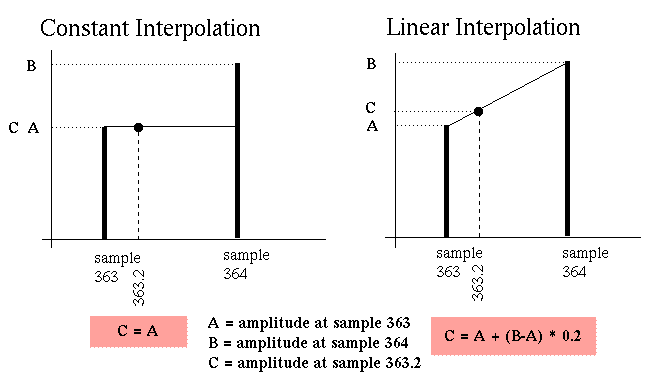6. Filter types: What is the difference between a linear and nonlinear filter? In other words, how does each type of filter affect the frequencies of the input signal?
 Answer: A Linear filter can only change the amplitudes of the input sound, either making them louder or softer. It cannot create new frequencies which are not present (have an amplitude of 0) in the input sound. A non-linear filter may generate new frequencies which are not the same as the frequencies in the input sound to the filter.
7. Filter types (2): Name two linear filters we covered in class. Name two non-linear filters we covered in class.
 Answer: linear filters: averaging filter, comb filter, DC-blocking filter, one-pole filter, one-zero filter, allpass filter, resonating filter, bi-quad filter, reverberation, etc. non-linear filters: ring modulation, FM synthesis, granular synthesis, transposing wavetable synthesis.
8. Linear filters: What are the three mathematical operations which can be done on a signal in a linear filter?
 Answer: add: two signals may be added together. scale: a signal may be scaled by a constant value to increase or decrease its amplitude. delay: a signal may be delayed in time. Note that none of these operations will add new frequencies to an input sound. One operation which is not allowed: multiplication: two signal cannot be multiplied together in linear filters (this is ring modulation).
9. Linear filters (2): Draw the flow-graph schematic of a generalized linear filter.
 input signal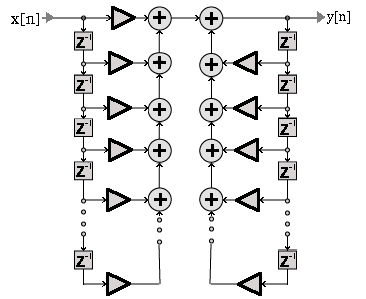output signal

All linear filters can be put into this form wich is useful for determining the difference equation of the filter.

10. Reverberation: Is reverberation a linear or non-linear filter? Why?
 Answer: Reverberation is a linear filter because no new frequencies are created by the reverberation (your voice does not change pitch depending on what type of room you are in does it?). The elements in a reverberator are scaling (sound hitting a wall and losing energy), adding (sound from different walls mix together), and delay (reflections of sound from different walls take different amounts of time to get to your ear). Since only these three types of filter elements are needed for reverberation, you have the same filter elements that linear filters are created from.
11. Filters: Explain these terms: "flowgraph", "difference equation", "transfer function", "frequency response", "pole-zero diagram". How do these terms relate to filters? How do these terms relate to each other? Only a top-level (i.e., vague) description is necessary, and no mathematics are involved in the explanation.
 Answer: a flowgraph describes how the sound flows through a filter. It is exactly like the objects in a Max/MSP audio patch. a difference equation is equivalent to a flowgraph. It demonstrates how to implement a filter in a program using a programming language such as C, C++, matlab or Java. a transfer function can be derived from the difference equation by taking the z-transform of the difference equation: replace all ys with Ys and xs with Xs and all [n-x]s with z^(-x)s. With a transfer function you can draw a pole-zero diagram. a pole-zero diagram indicate where the transfer function goes to zero or to infinity. Zeros are indicated on a complex plane as zeros, and infinities are represented with x's. All of these terms are different ways to describe how a (linear) filter affects the frequencies of an input sound. If you have the description of a filter in one of these forms, you can calculate all other forms of a filter description. Here is a road-map of the relationship between the terms:12. Extra Credit: If you were to implement a filter in a graphical environment such as Max/MSP, which of the terms in the previous question are relevant? If you were to implement a filter directly in C, which of the terms in the previous question would be relevant?
 Answer: The flowgraph is the most direct implementation of a filter in Max/MSP. The difference equation is the most relevant description of a filter for implementing in the C language.
13. Filters 2: What is the difference equation for the averaging filter (a filter which takes the current sample and the last sample and outputs the average of the two samples)? Draw the spectrum for the averaging filter.
 Answer: The difference equation for an averaging filter is: y[n] = (x[n] + x[n-1])/2. Below is the spectrum of the averaging filter. The averaging filter will have no effect on a 0 Hz signal (try this 0 Hz signal in the averaging filter: {1, 1, 1, 1, 1, 1, 1, 1, 1, 1, 1, 1, 1...}). The filter will completely remove any signal at 1/2 of the sampling rate (try this fs/2 Hz signal in the averaging filter: 1, -1, 1, -1, 1, -1, 1, -1, 1, -1, 1, -1, 1, -1}). For intermediate frequencies, the filter will affect the signal somewhat at low frequencies and will affect the signal greatly closer to fs/2 (half the sampling rate). In drawing the spectrum, you should note that at 0 Hertz, the averaging filter does not affect the input signal. At fs/2 the averaging filter will make the output of the filter all zeros. For frequencies inbetween 0 and fs/2, there will be approximately a straight line in the picture of the spectrum. Here is a picture of the exact spectrum generated by the averaging filter: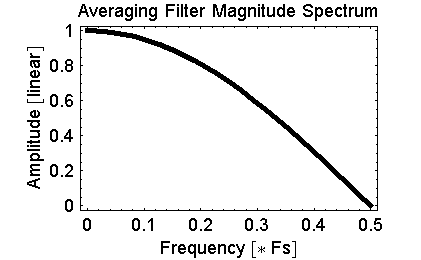Here is the Mathematica code used to generate the above spectrum plot: ```H[freq_] := 1/2 + 1/(2 E^(I 2 Pi freq) Plot[Abs[H[f]], {f, 0, 1/2}]; ```
14. Extra Credit: Draw the pole-zero diagram for the averaging filter.
 Answer: The pole zero diagram can be drawn by examining the transfer function of the averaging filter: Y/X = (1 + z)/2z . Where the function Y/X goes to zero, place a zero on the pole-zero diagram. This occurs when z = -1: ``` Y/X = (z + 1) / 2z = (-1 + 1) / -2 = 0 ``` There are no poles in the averaging filter because there are no values of z which will cause Y/X to go to infinity. Compare the pole-zero plot below with the spectrum of the averaging filter in the previous question. Can you see how the spectrum is derived from this pole-zero diagram?15. Pole-Zero Diagram: Draw the qualitative (approximate) spectrum (from 0 Hz to half the sampling rate) for the following pole-zero diagram: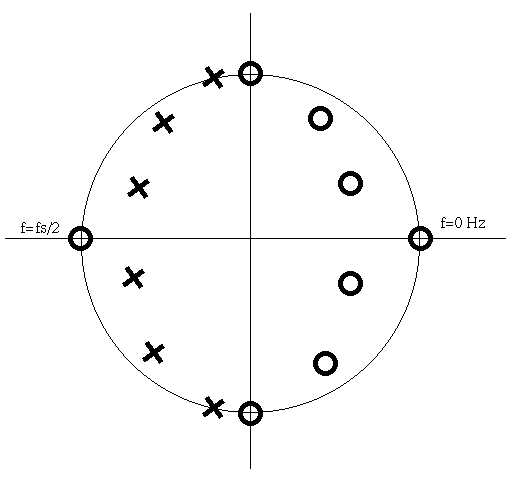Answer: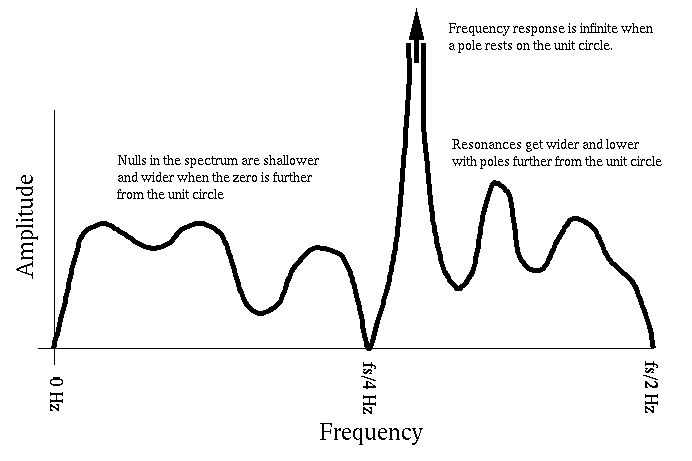16. Sinewaves and Hearing: What is the mathematical equation for a sinusoid? What are the three physical variables in the equation? How does these three variables relate to hearing?
Answer: Mathematical definition of a sinewave: A sin(2 pi f t + phi)  symbol physical meaning perceptual meaning A amplitude loudness f frequency pitch phi Phase Cannot be heard under normal monaural conditions (can be heard when it causes instantaneous amplitudes above the RMS amplitude of the signal). Can also be perceived as spatial localization on the horizontal plane of the ears.
17. Sinewaves and Mathematics: What is the mathematical definition of a complex sinusoid?
 Answer: Euler's identity demonstrates two methods of calculating a complex sinusoid: ``` e^(i x) = cos(x) + i sin(x) ``` The complex sinusoid can be thought of as a two-dimensional sinusoid. One dimension contains a cosine wave, and the perpendicular dimension contains a sinewave. Here is a picture of a complex sinusoid with the third dimension being time: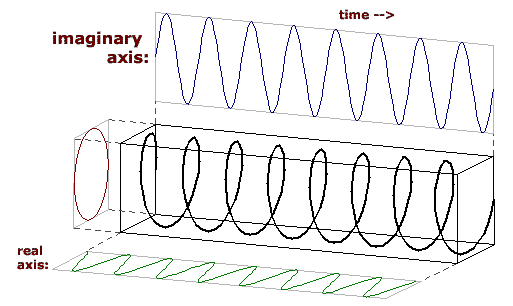18. Spectrum: What are the TWO steps that must be done to measure the amplitude of a sinusoid in an audio signal?
 Answer: Multiple the signal by a test sinusoid which has the same frequency as the one you are searching for the amplitude of in the test signal. Add the resulting signal, element by element to get the amplitude of the frequency. An optional third step is to normalize the amplitude. These steps work for complex sinusoids in all situations, but only work with real sinusoids in specific cases, since sinewaves cannot see cosinewaves, and vice versa.
19. Spectrum (2): How can the amplitude of a sinusoid with an arbitrary phase be measured in an audio signal?
 Answer: let As = amplitude of a test sinewave with frequency f. let Ac = amplitude of a test cosinewave with frequency f. let A = amplitude of a sinewave with frequency f and phase phi Then: A = sqrt( As * As + Ac * Ac)
20. Spectrum (3): Here is an audio signal:
```sample:  1     2      3     4      5     6     7      8      9     10
signal:  4.28, 0.34, -0.48, 3.91, -7.82, 2.55, 4.06, -5.50, -0.03, -1.30
```
Here is a picture of the signal (which repeats to the left and the right):And here is a test sinewave, and a test cosinewave:
```sample:  1    2      3      4      5     6    7      8      9     10
sine:    0,   0.95,  0.59, -0.59, -0.95, 0,   0.95,  0.59, -0.59, -0.95
cosine:  1,   0.31, -0.81, -0.81,  0.31, 1,   0.31, -0.81, -0.81,  0.31
```

Here is a picture of the test sinewave:Here is a picture of the test cosinewave: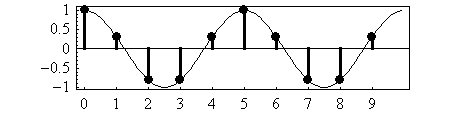(A) What is the amplitude of the test sinewave present in the signal? (B) What is the amplitude of the test sinewave present in the signal? (C) What is the amplitude of the sinusoid in the audio signal which has the same frequency as the test sine and cosine? (The normalization factor will be 5, so divided by 5 to find the final amplitude of the sinusoids, or don't worry about normalization).
 Answer: A: calculate the amplitude of the sinewave in the signal: ```sample: 1 2 3 4 5 6 7 8 9 10 signal: 4.28, 0.34, -0.48, 3.91, -7.82, 2.55, 4.06, -5.50, -0.03, -1.30 sine: 0, 0.95, 0.59,-0.59, -0.95, 0, 0.95, 0.59, -0.59, -0.95 ------------------------------------------------------------------ multiply: 0 +0.32 - 0.28 - 2.3 + 7.4 + 0 + 3.9 - 3.2 + 0.018 + 1.2 add: 7.037 = amplitude of the sinewave in the signal (normalize): 1.41 = normalized amplitude of the sinewave in the signal ``` B: calculate the amplitude of the cosinewave in the signal: ```sample: 1 2 3 4 5 6 7 8 9 10 signal: 4.28, 0.34, -0.48, 3.91, -7.82, 2.55, 4.06, -5.50, -0.03, -1.30 cosine: 1, 0.31, -0.81, -0.81, 0.31, 1, 0.31, -0.81, -0.81, 0.31 ------------------------------------------------------------------ multiply: 4.3 + 0.1 + 0.4 - 3.2 - 2.4 + 2.6 + 1.3 + 4.4 + 0.2 - 0.4 add: 7.068 = amplitude of the cosinewave in the signal (normalize): 1.41 = normalized amplitude of the cosinewave in the signal ``` C: calculate the amplitude of the unknown phased sinusoid in the signal which has the same frequency as the sine and cosine waves by using information from the previous question: ``` amplitude = sqrt(sineamp^2 + cosineamp^2) = sqrt( 1.41^2 + 1.41^2) = 2.0 ``` You could also have calculated the amplitude before normalizing the amplitudes of the sine and cosine test signals.
21. Extra Credit: What is the phase of the sinusoid in the signal in the previous question which you just calculated the amplitude of?
 Answer: Notice that the amplitudes of the sine and cosine in the previous problem were (approximately) equal to 1.41. The unknown phase of the sinusoid in the signal can be calculated by knowledge of the amplitudes of the sine and cosine test signals. The sine and cosine test signals form a complex sinusoid when you stick an i in front of the sine. The phase is the angle at which the amplitude of the sine (on y-axis) and cosine (x-axis) match. Here is how the phase of the sinusoid at the given frequency is calculated: ``` phase = arctangent(1.41/1.41) = arctangent(1) = pi/4 = 45 degrees ``` 100 bonus points if you were able to figure out that one :-).
22. Ring modulation: If the input signal into a ring modulator is a 500 Hz sinewave and the modulator is a 60 Hz sinewave, what are the output frequencies of the ring modulation that you will hear?
 Answer: There will be two frequencies in the output: 500 + 60 = 560 Hz and 500 - 60 = 440 Hz.
23. FM Modulation: What is the mathematical equation for FM Synthesis? What are the interesting control variables in the equation?
 Answer: Equation for simple FM synthesis is: ``` A sin(2 pi c t + I sin(2 pi m t)) ``` There are four interesting control variables in the equation: A -- The overall amplitude of the sound. c -- The carrier frequency. I -- The index of modulation (amplitude of the modulating sinewave). m -- The modulator frequency. Sidebands are generated in +/- m hertz steps from the carrier frequency.
24. FM Modulation (2): If the carrier is 400 Hz, and the modulator is 100 Hz, and the index of modulation is 3, what is the pitch of the resulting sound? Listen to the output of your lab program if you don't know.
 Answer: Here are the frequencies which will be generated with the given settings: ```400 Hz 500Hz 600Hz 700Hz 800Hz 900Hz (positive sideband) 300Hz 200Hz 100Hz 0Hz -100Hz (negative sideband) ``` This will create a harmonic spectrum for a note with a pitch at 100 Hz. Here is the actual spectrum which is generated: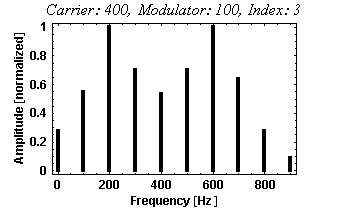25. Wavelength and Frequency: You thought you would never see a question like this again, but... Bats (depending on the species) can hear up to 150 kHz. What is the size of the smallest bug a bat can catch? Assume that they are using 100 kHz to echolocate their prey, and it takes 3 wavelengths of that frequency to detect reflections of the sound off of the bug, and the speed of sound in air is 350 meters/sec.
 Answer: ``` 350 m/s ---------- * 3 = 1.05 centimeters 100,000/s ```
26. Extra Credit: Why do I say that the bat's prey must be 3 times bigger than the detecting frequency? Larger bats use lower frequencies than smaller bats for finding prey, why might that be a reasonable situation?
 Answer: Bugs which are as large as one sound wavelength will not reflect that wavelength very well. It has to be a little larger in order to reflect sound back to the bat (unless the bat is very close). Larger bats need to eat larger bugs to stay alive (or they only eat fruit, so they just need echolocation to avoid hitting trees). Since they need to eat larger bugs, they do not need to hear as high as smaller bats in order to catch a meal.

--solutions by Craig Stuart Sapp